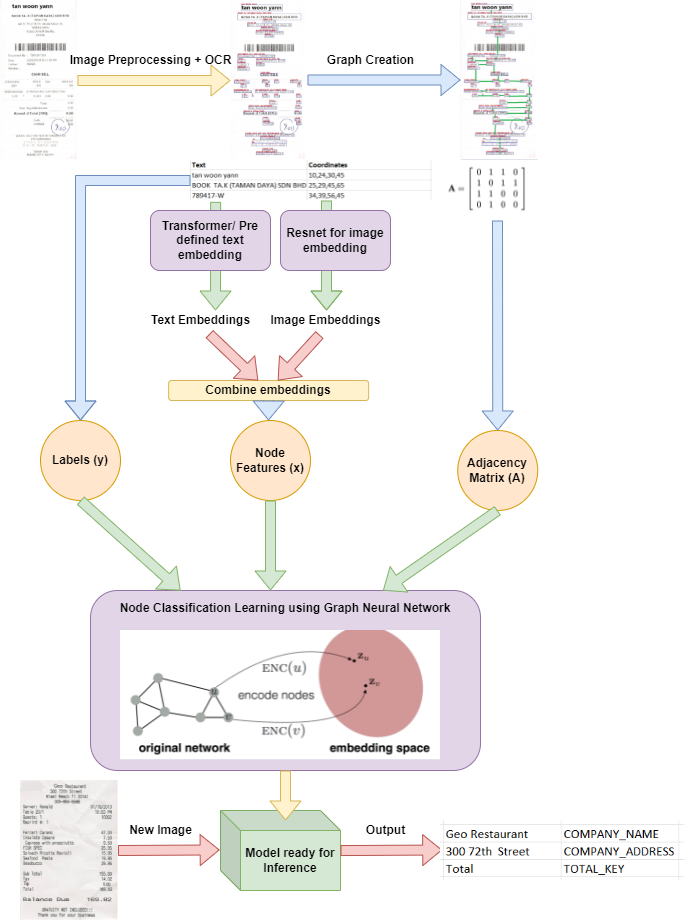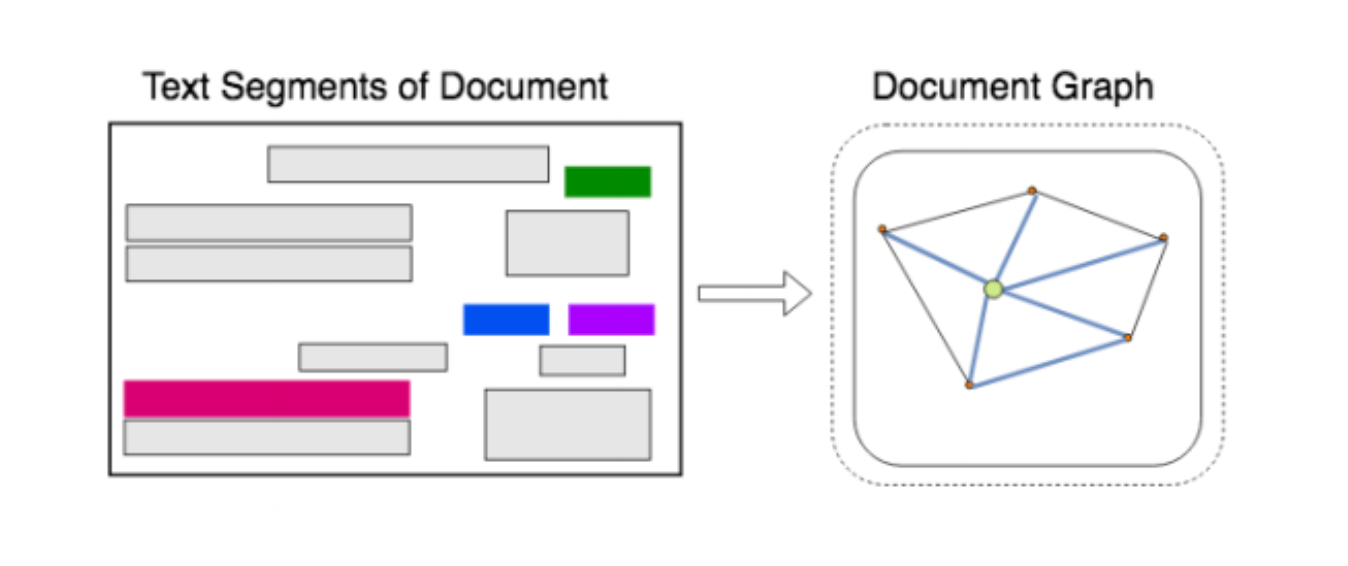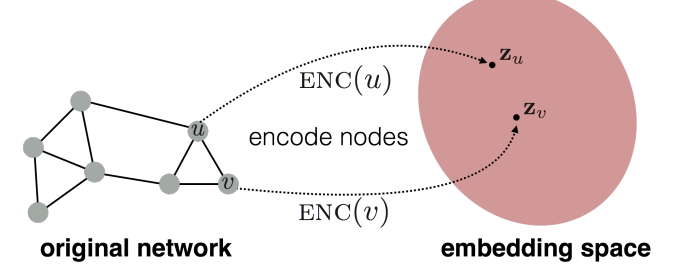# How Graph Neural Networks are used for Information Extraction?Fig 1. Basic common pipleine from receipt digitization

# Motivation to use GNN/GCN ?

The need to recognize local patterns in graphs, similarly to the way a CNN scans the input data through a small window recognizing local relations between the nodes within the window, a GCN could start by capturing local patterns between neighboring nodes in a graph . Recognizing hierarchies of patterns can be greatly exploited by GCNs.

# Pipeline Understanding

Let us try to understand the basic common pipeline for these kinds of projects:

• Ones the image is cropped and proprocessed accordingly, we provide this image to OCR  systems. You can use Google’s cloud APIs , Tesseract  or any OCR system of your choice in your budget, needs and system accuracy.
• After the process of OCR, we have a table which contains the text and their position in the input images. Usually OCR system provides the coordinates of left top point and right bottom point for each detected text.
• Now comes the interesting part, the outputs of OCR i.e the bounding boxes on receipts are used to create the input graph which will be used by graph neural networks. Each text/bounding box is considered to be a node, the edge connection creation can have multiple ways. One of such techniques  creates a maximum of four edges for each node, the edges connect each text area to its closest four neighboring text areas in each direction (Up, Down, Left and Right). Get some idea how this can be coded from here .Fig 2. Graph creation using bounding boxes from OCR
• These two type of embeddings are combined to create a new fusion embedding for better understanding of data and used as node inputs for Graph neural nets. To better understand the use of embedding it is suggested to go through this paper ones  and its implementation .
• For each ouput text we already have their output classes assigned to them, which will be used for learning. You can search for these kinds of receipts based datasets, one such is .
• At this point we have or adjacency matrix (A), feature matrix (x) created using the combination of word and image embeddings for each nodes and finnaly the labels (y). Now we can treat this as a normal machine learning problem where A and x are independent features and y is dependent which needs to be learned and predicted.
• A, x and y will be used to train a graph based neural network models which will learn to classify each node in the possible classes. The GCN, Graph Convolution Neural Network learns to embed node feature vector (combination of word embedding and the connection structure to other nodes) by generating a vector of real numbers that represents the input node as a point in an N-dimensional space, and similar nodes will be mapped to close neighboring points in the embedding space, allowing to train a model able to classify the nodes . This article depects theory related to node classification .Fig 3. Node classification using graph embedding space technique learned using a graph based neural net model

# References:

1. Image segmentation by OpenCV : https://www.kaggle.com/dmitryyemelyanov/receipt-ocr-part-1-image-segmentation-by-opencv
2. Pre-Processing from OCR!!! : https://towardsdatascience.com/pre-processing-in-ocr-fc231c6035a7
3. Optical Character Recognization : https://en.wikipedia.org/wiki/Optical_character_recognition
5. Tesseract : https://github.com/tesseract-ocr/tesseract
6. Effecient, Lexicon free OCR using deep learning : https://arxiv.org/abs/1906.01969
7. Information Extraction from Receipts with Graph Convolutional Networks : https://nanonets.com/blog/information-extraction-graph-convolutional-networks/
8. Graph Convolution on Structured Document : https://github.com/dhavalpotdar/Graph-Convolution-on-Structured-Documents/blob/master/grapher.py
9. PICK : https://arxiv.org/abs/2004.07464
10. PICK-pytorch : https://github.com/wenwenyu/PICK-pytorch
11. CORD : https://github.com/clovaai/cord
12. Automizing Receipt Digitization with OCR and Deep Learning : https://nanonets.com/blog/receipt-ocr/
13. Graph Convolution for Multimodel Information Extraction for Visually Rich Documents : https://arxiv.org/abs/1903.11279
14. Spektral : https://graphneural.network/
15. Understanding GCN for Node Classifcation : https://towardsdatascience.com/understanding-graph-convolutional-networks-for-node-classification-a2bfdb7aba7b
16. Extracting Structred Data from Invoices : https://medium.com/analytics-vidhya/extracting-structured-data-from-invoice-96cf5e548e40

--

--